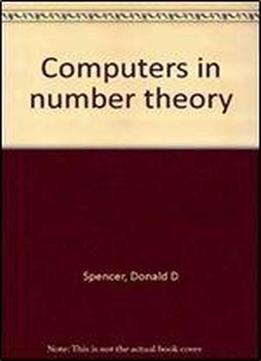# Computers In Number Theory by Donald D Spencer / 1982 / English / PDF

The computer is one of the most important calculating tools available to mathematicians. Today its use is quite commonplace, and for a mathemati­cian, the ability to write and understand computer programs is becoming as necessary as the ability to use a hand calculator. Many complex mathematical problems, as well as those of a more routine nature, can be solved conven­iently with a computer. Moreover, the search for solutions to research prob­lems is greatly aided by computers. Many methods of calculation that are complex or tedious when done by hand can be accomplished with ease on a computer. Computational techniques such as iteration methods are feasible when a computer is used to accomplish the calculations. In fact, many of the useful methods of numerical analysis can be employed by a mathematician who knows computer programming and has the use of a computer system.

views: 369